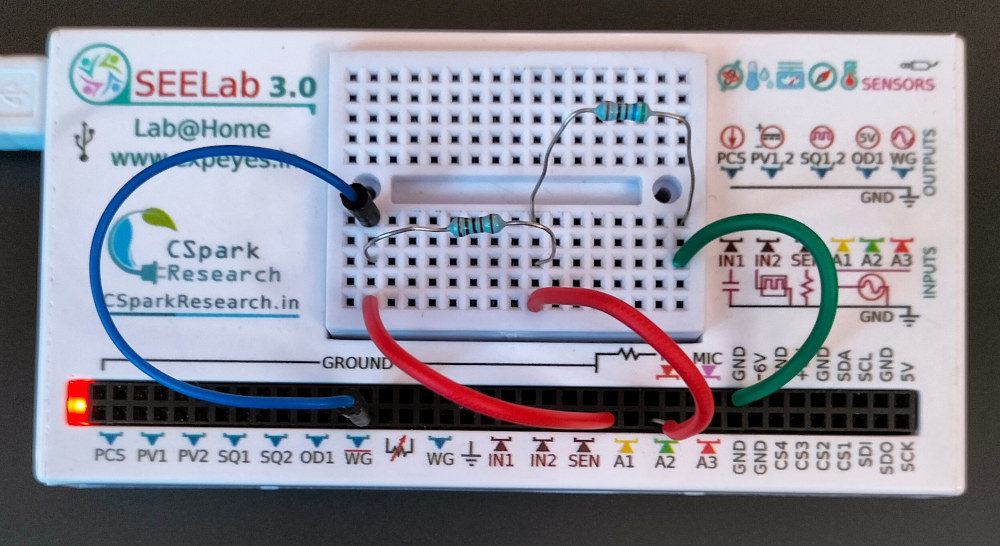# ExpEYES

## Resistor in AC Circuits

The objective is to explore the phase and amplitude relationships between the current and the voltage of an AC signal applied to a resistor. The method of current measurement is explained in the section on Ohm’s Law. In this experiment, the 1000 Ohm resistor and A2 forms the ammeter. Voltage across R1 is taken as the current waveform. This is plotted along with the voltage across R2.

• Connect R2 and R1 in series, between WG and Ground.
• Connect A1 to WG
• Connect A2 to the junction
• Select a region of the graph, so that the amplitudes of current and voltage are displayed.
• Calculate the value of R2, from the current and the voltage across R2.By selecting a region of the graph, the graph is mathematically analyzed to obtain the Peak voltage, frequency and the phase difference between the traces. We assume that the same amount of current flows through both R1 and R2 (neglecting the 1M input impedance of A2).

from the results

• Amplitude of current = 2.033 mA
• Amplitude of voltage across R2 = 1.141 V
• Current and Voltage are in phase

The RMS values of the voltages measured by A1 and A2 are displayed on the icons. In the case of a sine wave, the RMS value is obtained by dividing the peak value by $\sqrt{2}$. The displayed RMS value is calculated from the instantaneous values for a full cycle, by summing the squares of them and then taking the square root.